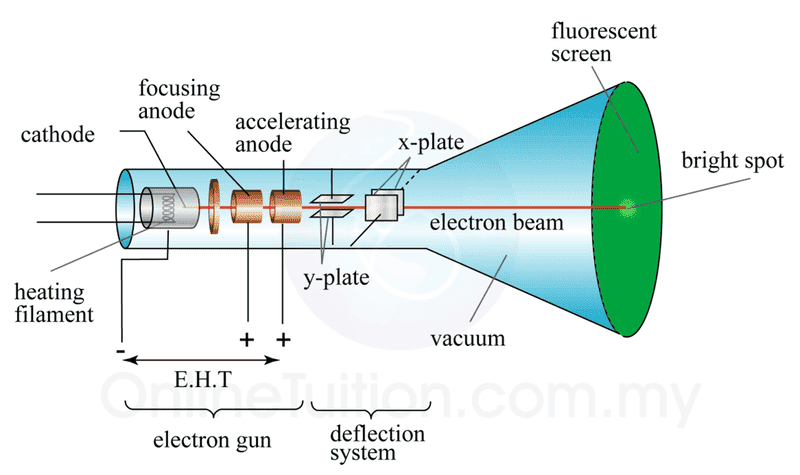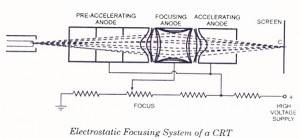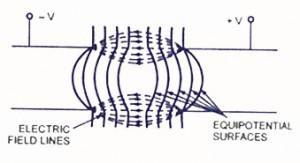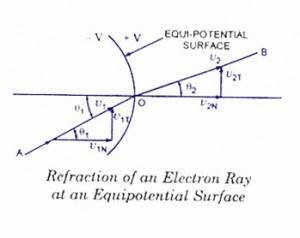# Collimation of an Electron Beam by the Anode of a CRT

Please help me in understanding the function of anode. As in image attached you see, once the electron beam is emitted from cathode and filtered by grid, it enters into the one or series of anodes. As anode is positively charged, so, to my understanding it has no electric field inside its hollow space. If the electron beam enters into this region, it should have experienced any force. Then how is beam focused? Please help me and oblige. High regards#### Attachments

BvU
Homework Helper
Google electrostatic focusing of electron beam

Google electrostatic focusing of electron beam

Delta2
Homework Helper
Gold Member
There is electric field inside each of the prefocusing, focusing and accelerating anode. That is because each anode is at a different potential, so there is electric field in the space between those three anodes, and this electric field fringes into the hollow space of each anode.

BvU
Homework Helper
Do you want me to click on the link for you ? What is unclear in the explanation
Electrostatic Focusing SystemElectrostatic Focusing

An electrostatic focusing system is shown in figure. Electrostatic lens consists of three anodes, with the middle anode at a lower potential than the other two electrodes.

In figure two anodes and its electrostatic lines and equipotential surfaces are shown. A pd is kept between these two electrodes so that an electric field is generated between them. Spreading of electric field is caused because of repulsion between electric lines. If equipotential lines are drawn, as shown in figure, they would bulge at the centre of the two anodes. As we know that electrons move in a direction opposite to that of electric field lines and equipotential surfaces are perpendicular to the electric field lines so force on the electron is exerted in the direction normal to the equipotential surface.Electrons entering at the centre line of the two anodes experience no force but electrons displaced from the centre line experience a force normal to the direction of equipotential surface and deflect, as shown in figure. An equi potential surface is shown, in which an electron with velocity V1 and at an angle θ. to the normal of equipotential surface enters and experiences a force in a direction normal to the equipotential surface. Thus the velocity of the electron increases to V2. This force on the electron is exerted in the direction normal to equipotential surface so only the normal component of electron velocity V1N increases to V2N and the tangential component of velocity V1T remains the same.From figure

V1T = V1 sin θ1,

V2T = V2 sin θ2

But V1T = V2T

V1 sin θ1 = V2 sin θ2

Or V2/V1 = sin θ1 / sin θ2

From the above expression it is obvious that equipotential surface acts as a concave lens in geometrical optics. That is why, this focusing system is named as an electrostatic lens. Now if we go back and refer figure, it can be seen that because of middle anode to a lower potential, electron beam coming from the cathode and passing through the first concave electrostatic lens tends to become more aligned with the axis of CRT and when it enters at the second concave electrostatic lens, formed between two anodes at different potentials, it is focused at the phosphor screen. Focal length of the electrostatic lens can lie adjusted by varying potential of middle anode with respect to other two anodes. Thus electron beam can be made to focus at the screen very precisely.

#### Attachments

Delta2
Homework Helper
Gold Member
@BvU , something that I cannot infer directly from the article, is the electric field inside the hollow space of each anode zero (or almost zero)? So the focusing is actually happening in the two spaces in between the three anodes?

BvU
•